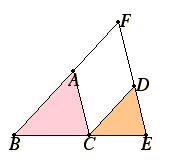# Proposition 4

In equiangular triangles the sides about the equal angles are proportional where the corresponding sides are opposite the equal angles.

Let ABC and DCE be equiangular triangles having the angle ABC equal to the angle DCE, the angle BAC equal to the angle CDE, and the angle ACB equal to the angle CED.

I say that in the triangles ABC and DEC the sides about the equal angles are proportional where the corresponding sides are opposite the equal angles.Let BC be placed in a straight line with CE.

Then, since the sum of the angles ABC and ACB is less than two right angles, and the angle ACB equals the angle DEC, therefore the sum of the angles ABC and DEC is less than two right angles. Therefore BA and ED, when produced, will meet. Let them be produced and meet at F.

I.28

Now, since the angle DCE equals the angle ABC, DC is parallel to FB. Again, since the angle ACB equals the angle DEC, AC is parallel to FE.

I.34

Therefore FACD is a parallelogram, therefore FA equals DC, and AC equals FD.

VI.2

And, since AC is parallel to a side FE of the triangle FBE, therefore BA is to AF as BC is to CE.

But FD equals AC, therefore BC is to CE as AC is to DE, and alternately BC is to CA as CE is to ED.

V.22

Since then it was proved that AB is to BC as DC is to CE, and BC is to CA as CE is to ED, therefore, ex aequali, BA is to AC as CD is to DE.

Therefore, in equiangular triangles the sides about the equal angles are proportional where the corresponding sides are opposite the equal angles.

Q.E.D.

## Guide

In the enunciation of this proposition the term “equiangular triangles” refers to two triangles whose corresponding angles are equal, not to two triangles each of which is equiangular (equilateral).

Euclid has placed the triangles in particular positions in order to employ this particular proof. Such positioning is common in Book VI and is easily justified.

This proposition implies that equiangular triangles are similar, a fact proved in detail in the proof of proposition VI.8. It also implies that triangles similar to the same triangle are similar to each other, also proved in detail in VI.8. The latter statement is generalized in VI.21 to rectilinear figures in general.

This proposition is frequently used in the rest of Book VI starting with the next proposition, its converse. It is also used in Books X through XIII.